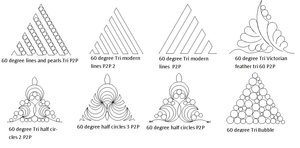# Triangles

Page 2 of 31
60 degree triangle setPrice: \$45.00
Machine Type
Page 2 of 31

## 60 Degree Triangle

A 60 Degree Triangle is one where all angles are the same and all sides are the same length.  It is also called an Equilateral Triangle.

## Flying Geese

Digitized Quilting Patterns for Flying Geese

## Half Square Triangle (HST)

The Half Square Triangle (HST) is one of the more basic units in quilting.  It is a triangle made from a perfect square cut on the diagonal.

All of our patterns that have "HST" in the name are shapes that have been made from a Half Square Triangle, ie; parallelogram, trapezoid, ect...

## Isosceles Triangles

Isosceles Triangles are ones that have have at least two equal sides

## Right Triangle

A Triangle where on corner is a Right Angle.  To use in opposite corners just Mirror the pattern